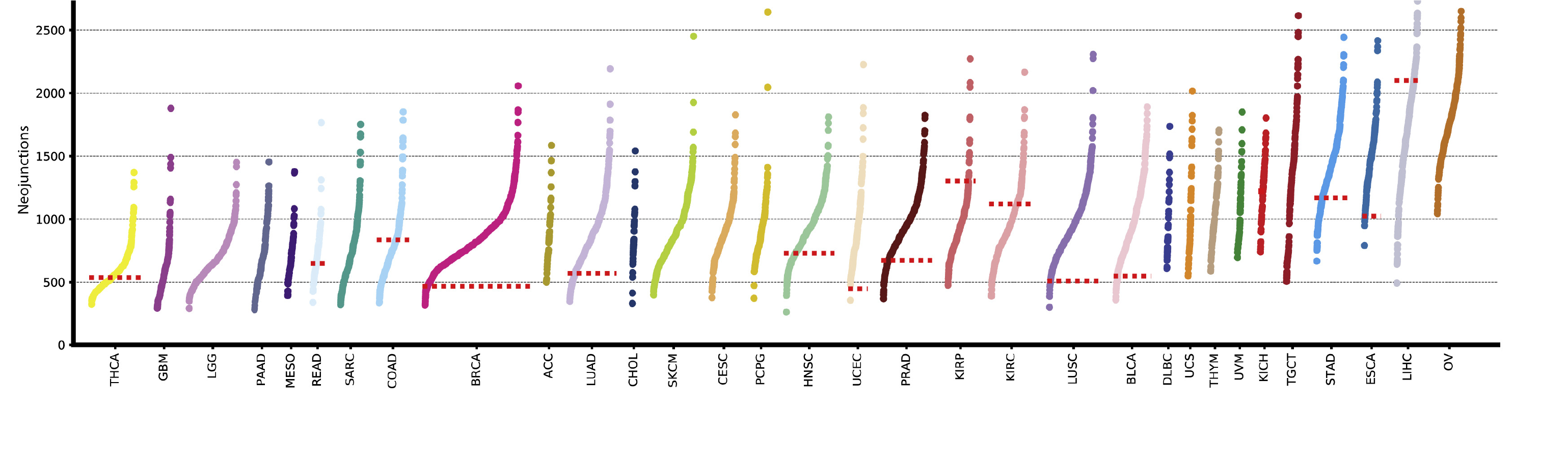Question: How to generate a stripplot like this in Python or R?
2
Ting-You Wang80 wrote:

How to generate a stripplot like this in Python or R?Thank you so much in advance.

R rna-seq visualization python • 530 views
modified 12 months ago by zx87549.1k • written 12 months ago by Ting-You Wang80

in R you can just make a scatter plot in something like ggplot2. You could use facets per gene.Another example is here. I don't think the author used facet to draw this. It seems that it used a special version of jitter (ordered).

1. do a box plot and jitter.
2. Do not draw box plot boundaries and let median line be there.
3. Do facet (or equivalent with mfrow) per gene
1

usually, jitter in boxplot only generates some noise in x-axis to avoid overlapping. I don't know how to do it in an ordered manner. Can you show some code snippet? Thank you.

Related post: Plots in R for bioinformatics analyses

1

some thing like this ?codeL

``````library(dplyr)
library(tidyr)
df=data.frame(s1=rnorm(1000,0,10), s2=rnorm(1000,1,10), s3=rnorm(1000,2,10))
library(ggplot2)
df1=gather(df,"sample","value")

ggplot(df1, aes(x = sample, y = value)) +
geom_point(aes(color=sample)) +
stat_summary(
fun.y = median,
fun.ymin = median,
fun.ymax = median,
geom = "crossbar",
color = "red",
width=0.2,
linetype = "dotted"
)+
ylim(-100,100)+
theme_bw()+
theme(legend.position="none")
``````